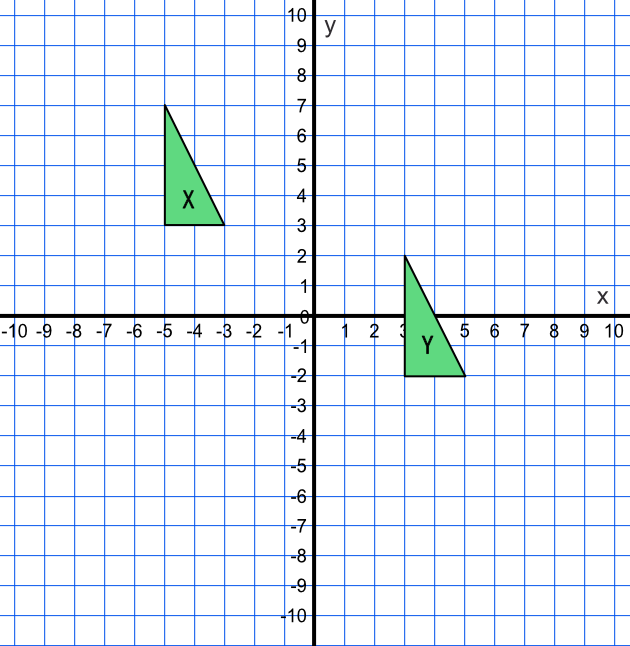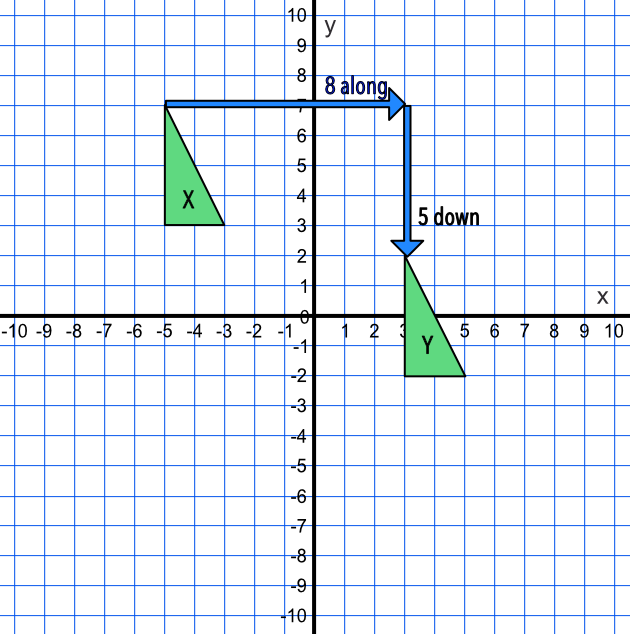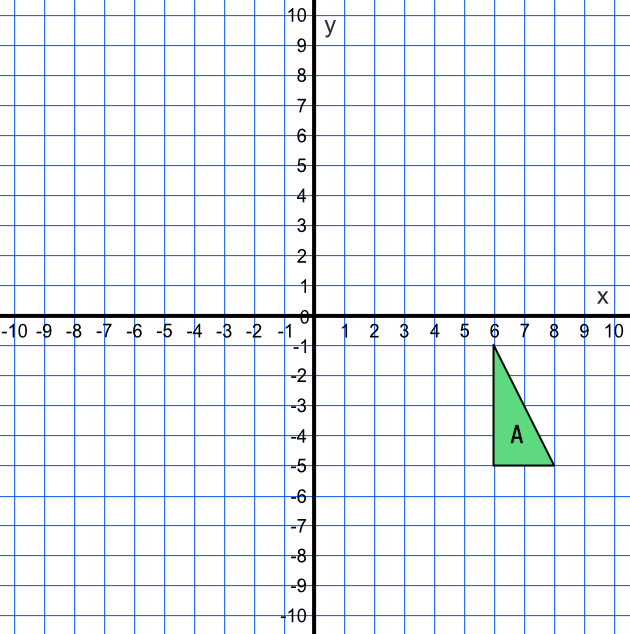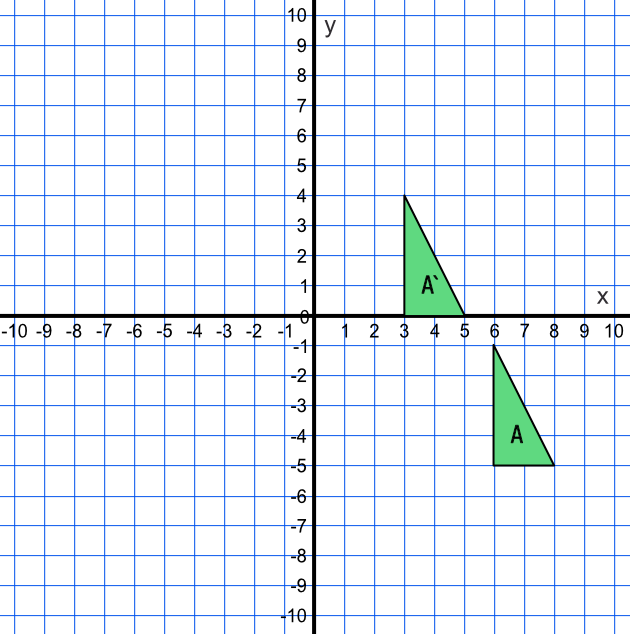Translation

## Translation

A Translation is a transformation that moves a shape. It does not turn, reflect or change the size of the shape.

Translations are given by a column vector. A column vector is a pair of numbers in brackets, one above the other.

The top number gives the movement in the horizontal, or x-direction, with positive values translating to the left. The lower number gives the movement in the vertical, or y-direction, with positive values translating up.

The vector is required to define a translation.

A translation preserves the angles and sizes of a shape.

## Example 1

What is the transformation that maps shape X onto shape Y?Choose a single vertex on the shape X, and determine how that point moves to the same vertex on shape Y.

The point moves 8 along, and 5 down.

This is a vector of ((8), (-5)). the down direction makes the Y-movement a negative value.Answer: ((8), (-5))

## Example 2

Translate the given shape A by a vector of ((-3), (5)).The top line of the vector is -3, so the shape will move 3 places to the left.

The bottom number is 5, and as this is positive move five places up.

Choose one vertex on the shape and move for that one vertex. The shape will move onto shape A`.